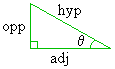# Math2.org Math Tables:(Math)
 Sine Topics overview formal definition graph properties expansions derivative integral Sine: Overview The sine (abbreviated "sin") is a type of trigonometric function. Definition 1 is the simplest and most intuitive definition of the sine function. It basically says that, on a right triangle, the following measurements are related: the length of the triangle's hypotenuse the length of one of the other sides the measurement of the angle (q) opposite to that other sideFuthermore, Definition I gives an exact equation that describes this relation: sin(q) = opposite / hypotenuse This equation says that if we evaluate the sine of that angle q, we will get the exact same value as if we divided the length of the side opposite to that angle by the length of the triangle's hypotenuse. The relation holds for any right triangle, regardless of size. The main result is this: If we know the values of any two of the above quantities, we can use the above relation to mathematically derive the third quantity. For example, the sine function allows us to answer any of the following three questions: "Given a right triangle, where the measurement of one of the non-right angles (q) is known and the length of the side opposite to that angle q is known, find the length of the triangle's hypotenuse." "Given a right triangle, where the measurement of one of the non-right angles (q) is known and the length of the triangle's hypotenuse is known, find the length of the side opposite to that angle q." "Given a right triangle, where the length of the triangle's hypotenuse and the length of one of the triangle's other sides is known, find the measurement of the angle (q) opposite to that other side." The function takes the form y = sin(q). Usually, q is an angle measurement and y denotes a length. The sine function, like all trig functions, evaluates differently depending on the units on q, such as degrees, radians, or grads. For example, sin(90°) = 1, while sin(90)=0.89399.... explaination